# Catch up on the latest AI articles# You Don't Need Big Computers Anymore To Train Deep Generative Models! Binary Deep Generative Models

3 main points
✔️ The first binary neural network is adopted as the architecture of the deep generative model, and the image is successfully generated.
✔️ To enjoy the effect of batch normalization used in conventional binary neural networks in deep generative models, we propose "Binary weight normalization" which is a binary version of weight normalization.
✔️ We succeeded in binary normalization of activation functions and residual couplings, which have been used as architectures for deep generative models, without loss of performance.

written by Thomas BirdFriso H. KingmaDavid Barber
(Submitted on 26 Oct 2020 (v1), last revised 3 May 2021 (this version, v2))

Subjects: Machine Learning (cs.LG)code：The images used in this article are from the paper, the introductory slides, or were created based on them.

## first of all

Deep models that generate high-dimensional data such as images require high expressive power, and training such deep generative models requires high computational cost.

In this paper, we use a binary neural network, which is an architecture in which the weights of the network are binary, to significantly reduce the computational cost without harming the performance of the model. In this paper, we briefly describe binary neural networks as a background and then introduce the method introduced in the paper in detail.

## What is a binary neural network?

A binary neural network is a neural network in which the weight of the network is expressed by the binary value of {-1, 1}. It becomes possible to hold the parameter which originally needed to be held by 32 bits by making the weighted binary by only 1 bit.

Not only that, there is a research report that the learning speed can be accelerated by 2 times just by making the weights binary, and if the input to the layer is limited to binary, the speed can be accelerated by 29 times further. Thus, the binary neural network is an excellent architecture from two viewpoints of memory efficiency and learning efficiency.

However, binarizing the weights also has drawbacks, such as reduced expressiveness of the model and difficulties in optimization.

Typically, in a binary neural network, binarization is performed on the weights and the inputs to each layer. To binarize the input to a layer, a sign function is applied to the output of the previous layer. The sign function refers to the function expressed by the following equation.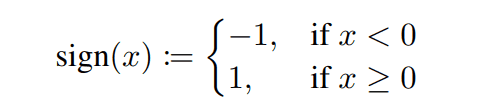For the binary weight parameter, a sign function is applied to the hidden parameter which is represented by a real value. In general, we do not optimize the binary weights directly during optimization but optimize this hidden parameter.

Gradient-based optimization methods are used in deep learning, but the sign function is difficult to learn because the slope is zero in most places. Therefore, we use a method called straight-through estimator (STE) to approximate it as follows.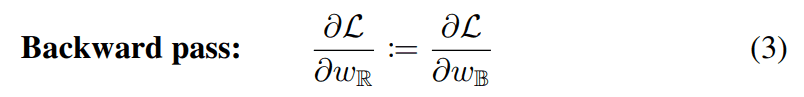It is known that the method of canceling the gradient when it becomes very large is effective, and following this, we can obtain the following clipped weight parameter update formula.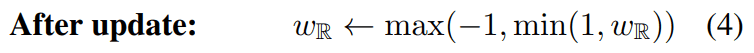## Targeted deep generative models

The deep generative models that have been implemented using the binary neural networks described above are Hierarchical VAE and Flow++. The detailed description of the models is left to other articles, but please note that the changes made by the binary neural networks described below do not change the purpose of each generative model, but are only architectural changes.

The binarization methods described below focus on "weight normalization" and "residual combination" used in deep generative models.

## binary deep generative model

In using binary neural networks for deep generative models, there are two binarization methods that this paper proposes.

They are "Binarization of weight normalization " and "Binarization of residual neural network" respectively. I will explain them one by one.

### Binarization of weight normalization

In deep generative models, weight normalization is often used instead of batch normalization. It is also used in Hierarchical VAE and Flow++ introduced above. The expression of weight normalization can be expressed as follows. Now, let's consider binarizing $v$ in the equation.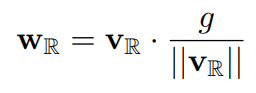When $v$ is binarized, the Euclidean norm in the equation can be written as the square root of the dimensionality $n$. In other words, it is not necessary to calculate the norm of the weights, and the calculation time can be shortened.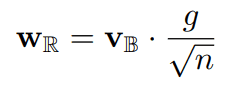Also, in binary weight normalization, the only actual operation performed is to multiply the coefficient $\alpha=gn^{-1/2}$. Therefore, the convolution operation with binary weight normalization corresponds to the operation of multiplying the coefficient $\alpha$ after the convolution with the binary weights.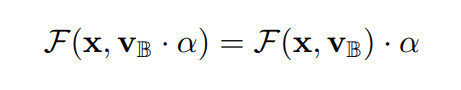### Binarization of residual neural networks

A structure that frequently appears in the architecture of deep generative models is the residual join.

Residual coupling is an architecture that has a skip connection structure as shown in the following equation. The residual coupling has been studied to improve the expressive power of the model without causing gradient vanishing, to solve the degradation problem that accuracy decreases as the layers become deeper.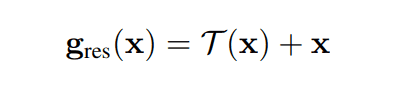The authors choose only residual bonds as the target of binarization and cite the following points as reasons.

• Generative models require particularly high expressive power, but in principle, binarization reduces the expressive power.
• In the generative model, the likelihood of a data point is sensitive to the output of the model, and the output of the model fluctuates greatly when it is binarized
• The fact that the property that the identity function is learnable is preserved even when the residual bound is binarized

In particular, for normalized flow-based generative models such as Flow++, it is important that the residual coupling used in the network can easily represent an isomorphic map since the function to be trained must have an inverse transformation.

The architecture of the residual coupling used in the actual experiment is as follows. There are two types, one with real vectors and the other with binary vectors, and ELU and sign functions are used as activation functions, respectively. The convolutional layer used in the conventional residual join is replaced by a BWN convolutional layer.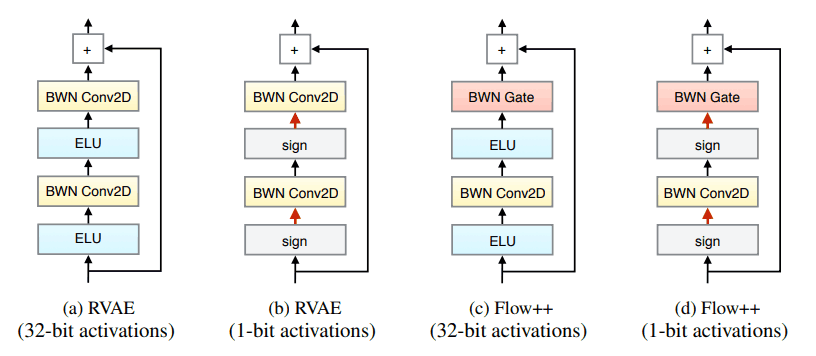## Image Generation Experiment

To confirm that deep generative models can be successfully trained using binary neural networks, we conducted experiments on image generation in CIFAR and ImageNet. We used two generative models, ResNet VAE and Flow++.

The following table compares the loss when training the model on each data set. In the table, 32 bits indicate the implementation with real numbers, and 1 bit indicates the implementation with binary numbers. In addition, the "increased width" item shows the results when the number of filters in ResNet is increased (from 256 to 336).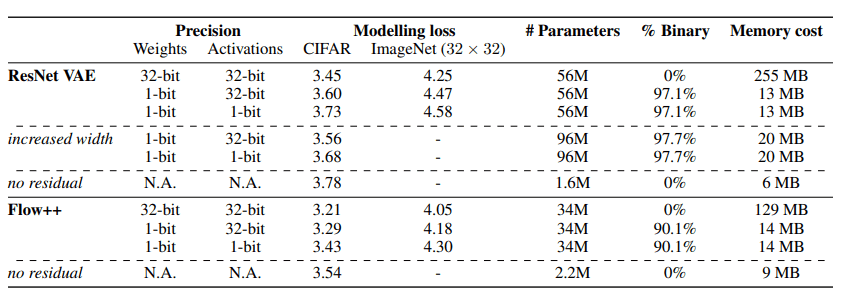The results of binarizing the weights in the residual join are slightly worse than those of using real weights. However, in both models, the memory cost is reduced from 1/10 to 1/20 by binarization.

This result shows that there is a trade-off between memory efficiency and performance by binarization.

The significant reduction in memory cost allows us to train models with larger network sizes, but as can be seen in the results for incremented width, we did not observe an improvement in performance by increasing the number of binary weights.

The actual generated image looks like the following. On the left is the conventional model using real weights, and on the right is the model using binary weights. It can be seen that the generated image of the binary model is not inferior to the conventional model.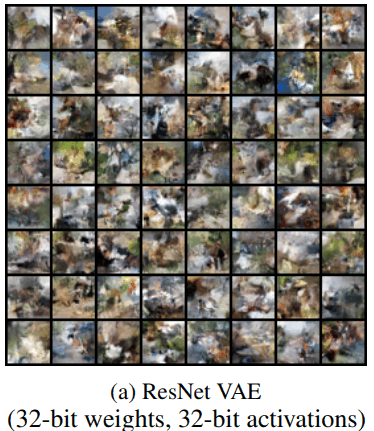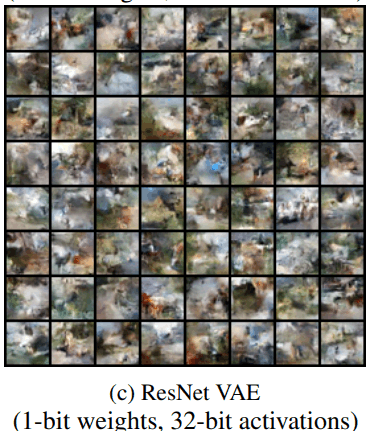## summary

How did you like it? The main purpose of this paper is simple: to improve memory efficiency in training deep generative models, but it was surprising that the memory cost is reduced to 1/10! From now on, it may be possible to train large-scale generative models on PCs and smartphones.

I hope that in the future there will be a binary neural network framework that can be used as stably as PyTorch or TensorFlow.

If you have any suggestions for improvement of the content of the article,